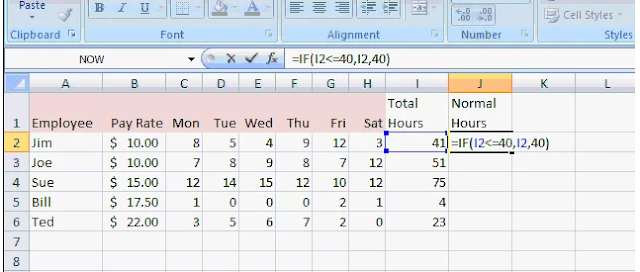Overtime pay is good incentive for employees. It will increase the your organisation's productivity because overtime pay encourages employees to spend more time for organisation's works. Recently, I appointed an employee part time for my Accounting Education. So, I am searching good excel formulas on the net for calculating overtime pay. I found a good excel training channel (name 599CD Computer Training) on the net who provided good formula for calculating overtime pay in excel. I am explaining these formulas stepwise.

1st Step : To Calculate total Hours of Work in a Week

Suppose, you have 5 employees who work different time in your organisation. Record their real working hours in a week. On these basis, you can calculate total hours of work in a week. In your case, you can also calculate total hours of work in a month by applying simple sum formula in excel.

=sum(number1,number2........)

2nd Step : To Calculate Normal Hours

By using logical formula, we can calculate normal hours of each employee from total working hours. Following is this formula.

=IF(logical_test;value_if_true;value_if_false)

Following is screenshot in which you see, how it has been used in real problem.

After calculating first value, we will drag this value and we will calculate all the normal hours values.

Condition of screenshot question : Those who work 40 hours or less get normal pay. From 40 to 50 hours will receive time-and-a-half, and over 50 hours earns an employee double-time.

3rd Step : To Calculate Overtime in Hours

We will also use logical formula when we will calculate overtime in hours.  Following is this formula.

=IF(logical_test;value_if_true;value_if_false)

4th Step : To Calculate Overtime Pay

Now, it will become so easy for you to calculate overtime pay. It is just multiplication of overtime hours and rate of salary. Following video will explain it in detail.

Related : Accounting in Excel Tips.

## \$type=three\$a=hide\$cm=hide\$s=hide\$show=/2019/06/10-steps-to-become-better-investor.html\$l=hide

Name

false
ltr
item
Accounting Education: How to Calculate Overtime Pay in Excel
How to Calculate Overtime Pay in Excel
http://1.bp.blogspot.com/-mJC_V6pHEoU/TsIu9mmcM0I/AAAAAAAAHDY/7UGiwguFyaI/s640/excel+formula.PNG
http://1.bp.blogspot.com/-mJC_V6pHEoU/TsIu9mmcM0I/AAAAAAAAHDY/7UGiwguFyaI/s72-c/excel+formula.PNG
Accounting Education
http://www.svtuition.org/2011/11/how-to-calculate-overtime-pay-in-excel.html
http://www.svtuition.org/
http://www.svtuition.org/
http://www.svtuition.org/2011/11/how-to-calculate-overtime-pay-in-excel.html
true
2410664366776677676
UTF-8